This book is archived and will be removed July 6, 2022. Please use the updated version.

Geometric Optics and Image Formation

# 16 The Simple Magnifier

### Learning Objectives

By the end of this section, you will be able to:

• Understand the optics of a simple magnifier
• Characterize the image created by a simple magnifier

The apparent size of an object perceived by the eye depends on the angle the object subtends from the eye. As shown in (Figure), the object at A subtends a larger angle from the eye than when it is position at point B. Thus, the object at A forms a larger image on the retina (see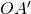) than when it is positioned at B (see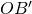). Thus, objects that subtend large angles from the eye appear larger because they form larger images on the retina.

Size perceived by an eye is determined by the angle subtended by the object. An image formed on the retina by an object at A is larger than an image formed on the retina by the same object positioned at B (compared image heightsto).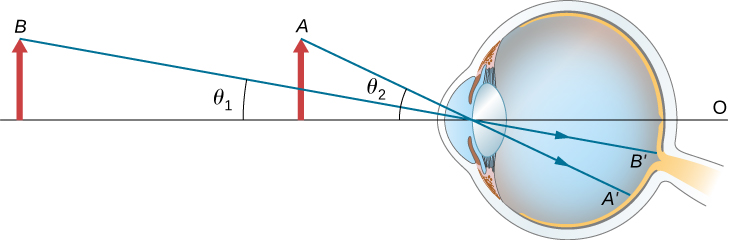We have seen that, when an object is placed within a focal length of a convex lens, its image is virtual, upright, and larger than the object (see part (b) of (Figure)). Thus, when such an image produced by a convex lens serves as the object for the eye, as shown in (Figure), the image on the retina is enlarged, because the image produced by the lens subtends a larger angle in the eye than does the object. A convex lens used for this purpose is called a magnifying glass or a simple magnifier.

The simple magnifier is a convex lens used to produce an enlarged image of an object on the retina. (a) With no convex lens, the object subtends an angle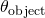from the eye. (b) With the convex lens in place, the image produced by the convex lens subtends an angle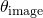from the eye, with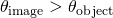. Thus, the image on the retina is larger with the convex lens in place.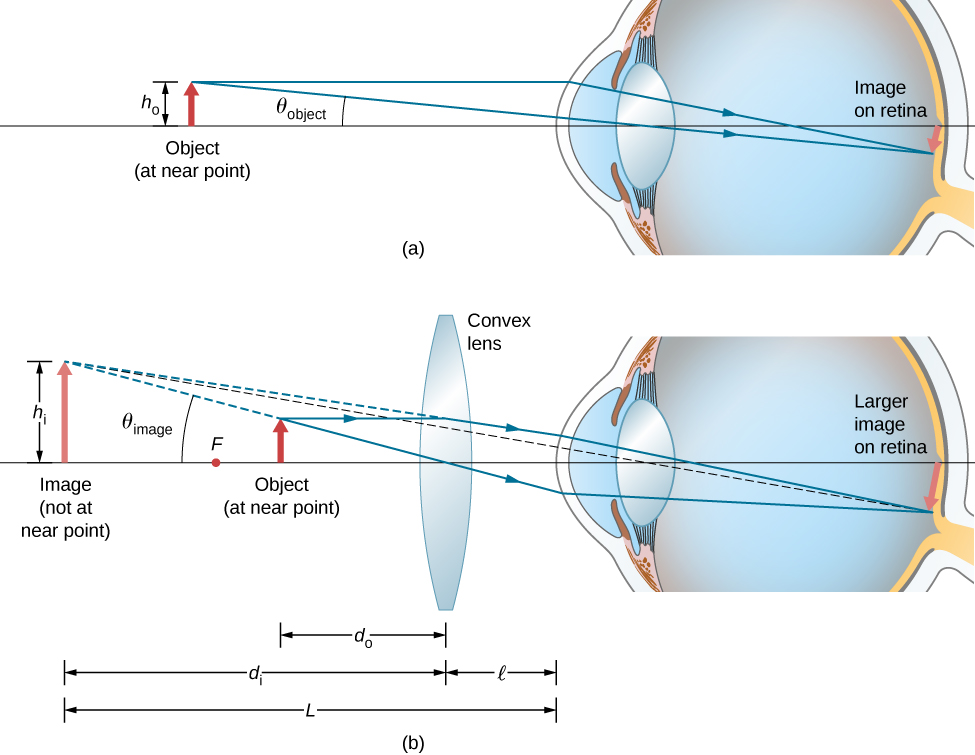To account for the magnification of a magnifying lens, we compare the angle subtended by the image (created by the lens) with the angle subtended by the object (viewed with no lens), as shown in (Figure). We assume that the object is situated at the near point of the eye, because this is the object distance at which the unaided eye can form the largest image on the retina. We will compare the magnified images created by a lens with this maximum image size for the unaided eye. The magnification of an image when observed by the eye is the angular magnification M, which is defined by the ratio of the anglesubtended by the image to the anglesubtended by the object: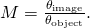Consider the situation shown in (Figure). The magnifying lens is held a distancefrom the eye, and the image produced by the magnifier forms a distance L from the eye. We want to calculate the angular magnification for any arbitrary L and. In the small-angle approximation, the angular sizeof the image is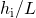. The angular sizeof the object at the near point is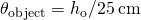. The angular magnification is then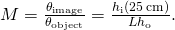Using (Figure) for linear magnification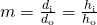and the thin-lens equation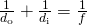in (Figure), we arrive at the following expression for the angular magnification of a magnifying lens: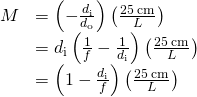From part (b) of the figure, we see that the absolute value of the image distance is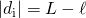. Note that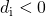because the image is virtual, so we can dispense with the absolute value by explicitly inserting the minus sign: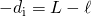. Inserting this into (Figure) gives us the final equation for the angular magnification of a magnifying lens: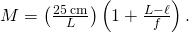Note that all the quantities in this equation have to be expressed in centimeters. Often, we want the image to be at the near-point distance (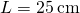) to get maximum magnification, and we hold the magnifying lens close to the eye (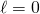). In this case, (Figure) gives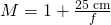which shows that the greatest magnification occurs for the lens with the shortest focal length. In addition, when the image is at the near-point distance and the lens is held close to the eye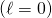, then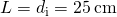and (Figure) becomes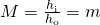where m is the linear magnification ((Figure)) derived for spherical mirrors and thin lenses. Another useful situation is when the image is at infinity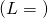. (Figure) then takes the form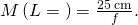The resulting magnification is simply the ratio of the near-point distance to the focal length of the magnifying lens, so a lens with a shorter focal length gives a stronger magnification. Although this magnification is smaller by 1 than the magnification obtained with the image at the near point, it provides for the most comfortable viewing conditions, because the eye is relaxed when viewing a distant object.

By comparing (Figure) with (Figure), we see that the range of angular magnification of a given converging lens is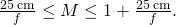Magnifying a Diamond A jeweler wishes to inspect a 3.0-mm-diameter diamond with a magnifier. The diamond is held at the jeweler’s near point (25 cm), and the jeweler holds the magnifying lens close to his eye.

(a) What should the focal length of the magnifying lens be to see a 15-mm-diameter image of the diamond?

(b) What should the focal length of the magnifying lens be to obtain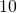magnification?

Strategy We need to determine the requisite magnification of the magnifier. Because the jeweler holds the magnifying lens close to his eye, we can use (Figure) to find the focal length of the magnifying lens.

Solution

1. The required linear magnification is the ratio of the desired image diameter to the diamond’s actual diameter ((Figure)). Because the jeweler holds the magnifying lens close to his eye and the image forms at his near point, the linear magnification is the same as the angular magnification, so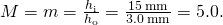The focal length f of the magnifying lens may be calculated by solving (Figure) for f, which gives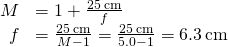2. To get an image magnified by a factor of ten, we again solve (Figure) for f, but this time we use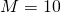. The result is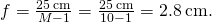Significance Note that a greater magnification is achieved by using a lens with a smaller focal length. We thus need to use a lens with radii of curvature that are less than a few centimeters and hold it very close to our eye. This is not very convenient. A compound microscope, explored in the following section, can overcome this drawback.

### Summary

• A simple magnifier is a converging lens and produces a magnified virtual image of an object located within the focal length of the lens.
• Angular magnification accounts for magnification of an image created by a magnifier. It is equal to the ratio of the angle subtended by the image to that subtended by the object when the object is observed by the unaided eye.
• Angular magnification is greater for magnifying lenses with smaller focal lengths.
• Simple magnifiers can produce as great as tenfold () magnification.

### Problems

If the image formed on the retina subtends an angle of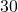and the object subtends an angle of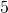, what is the magnification of the image?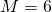What is the magnification of a magnifying lens with a focal length of 10 cm if it is held 3.0 cm from the eye and the object is 12 cm from the eye?

How far should you hold a 2.1 cm-focal length magnifying glass from an object to obtain a magnification of? Assume you place your eye 5.0 cm from the magnifying glass.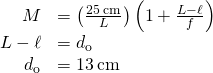You hold a 5.0 cm-focal length magnifying glass as close as possible to your eye. If you have a normal near point, what is the magnification?

You view a mountain with a magnifying glass of focal length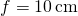. What is the magnification?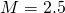You view an object by holding a 2.5 cm-focal length magnifying glass 10 cm away from it. How far from your eye should you hold the magnifying glass to obtain a magnification of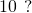A magnifying glass forms an image 10 cm on the opposite side of the lens from the object, which is 10 cm away. What is the magnification of this lens for a person with a normal near point if their eye 12 cm from the object?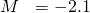An object viewed with the naked eye subtends a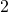angle. If you view the object through amagnifying glass, what angle is subtended by the image formed on your retina?

For a normal, relaxed eye, a magnifying glass produces an angular magnification of 4.0. What is the largest magnification possible with this magnifying glass?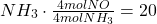What range of magnification is possible with a 7.0 cm-focal length converging lens?

A magnifying glass produces an angular magnification of 4.5 when used by a young person with a near point of 18 cm. What is the maximum angular magnification obtained by an older person with a near point of 45 cm?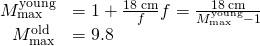### Glossary

angular magnification
ratio of the angle subtended by an object observed with a magnifier to that observed by the naked eye
simple magnifier (or magnifying glass)
converging lens that produces a virtual image of an object that is within the focal length of the lens